# Release Notes for v0.8.1

## It's 2020 Now

For the last release I forgot to change the copyright notice, that's fixed now:

```\$ ./target/release/rs_pbrt assets/scenes/cornell_box.pbrt
pbrt version 0.8.1 [Detected 28 cores]
Copyright (c) 2016-2020 Jan Douglas Bert Walter.
Rust code based on C++ code by Matt Pharr, Greg Humphreys, and Wenzel Jakob.
...
```

## Files Changed

```36 files changed, 354 insertions(+), 393 deletions(-)
...
deleted    examples/shapes_sphere_intersect.rs
...
```

## SurfaceInteraction

The biggest change of this release is about the struct `SurfaceInteraction`.

```modified   src/core/interaction.rs
@@ -274,7 +274,7 @@ pub struct SurfaceInteraction<'a> {
pub dvdx: Cell<Float>,
pub dudy: Cell<Float>,
pub dvdy: Cell<Float>,
-    pub primitive: Option<&'a Primitive>,
+    pub primitive: Option<*const Primitive>,
pub bsdf: Option<Bsdf>,
pub bssrdf: Option<TabulatedBssrdf>,
```

The change is less about the struct itself (only the optional pointer to a `Primitive` changed), but rather when the memory for an instance of the struct is allocated and how it is passed to other parts of the code to be either modified or simply used.

```modified   src/shapes/triangle.rs
@@ -148,7 +147,7 @@ impl Triangle {
self.mesh.p[self.mesh.vertex_indices[(self.id * 3) as usize + 2] as usize];
bnd3_union_pnt3(&Bounds3f::new(p0, p1), &p2)
}
-    pub fn intersect(&self, ray: &Ray) -> Option<(Rc<SurfaceInteraction>, Float)> {
+    pub fn intersect(&self, ray: &Ray, t_hit: &mut Float, isect: &mut SurfaceInteraction) -> bool {
// get triangle vertices in _p0_, _p1_, and _p2_
let p0: &Point3f = &self.mesh.p[self.mesh.vertex_indices[(self.id * 3) as usize] as usize];
let p1: &Point3f =
```

In the past the memory was allocated e.g. during a `Triangle` intersection with a `Ray`, now we follow more closely the C++ code, where the memory is allocated once e.g. before we ask for an intersection with the `Scene` and possibly re-used many times instead of re-allocating another `SurfaceInteraction` struct.

You can see the difference between v0.8.0 and v0.8.1 easily by running heaptrack.

### Ambient Occlusion

```modified   src/integrators/ao.rs
@@ -65,9 +64,10 @@ impl AOIntegrator {
differential: r.differential,
medium: r.medium.clone(),
};
-        if let Some(mut isect) = scene.intersect(&mut ray) {
+        let mut isect: SurfaceInteraction = SurfaceInteraction::default();
+        if scene.intersect(&mut ray, &mut isect) {
-            Rc::get_mut(&mut isect).unwrap().compute_scattering_functions(&ray, true, mode);
+            isect.compute_scattering_functions(&ray, true, mode);
// if (!isect.bsdf) {
//     VLOG(2) << "Skipping intersection due to null bsdf";
//     ray = isect.SpawnRay(ray.d);

```

For Ambient Occlusion you can see the effect between version 0.8.0 (left side) and 0.8.1 (right side) basically on the right column. That column is reduced a lot. The other columns are more or less the same, except that through scaling you see more details on the right side.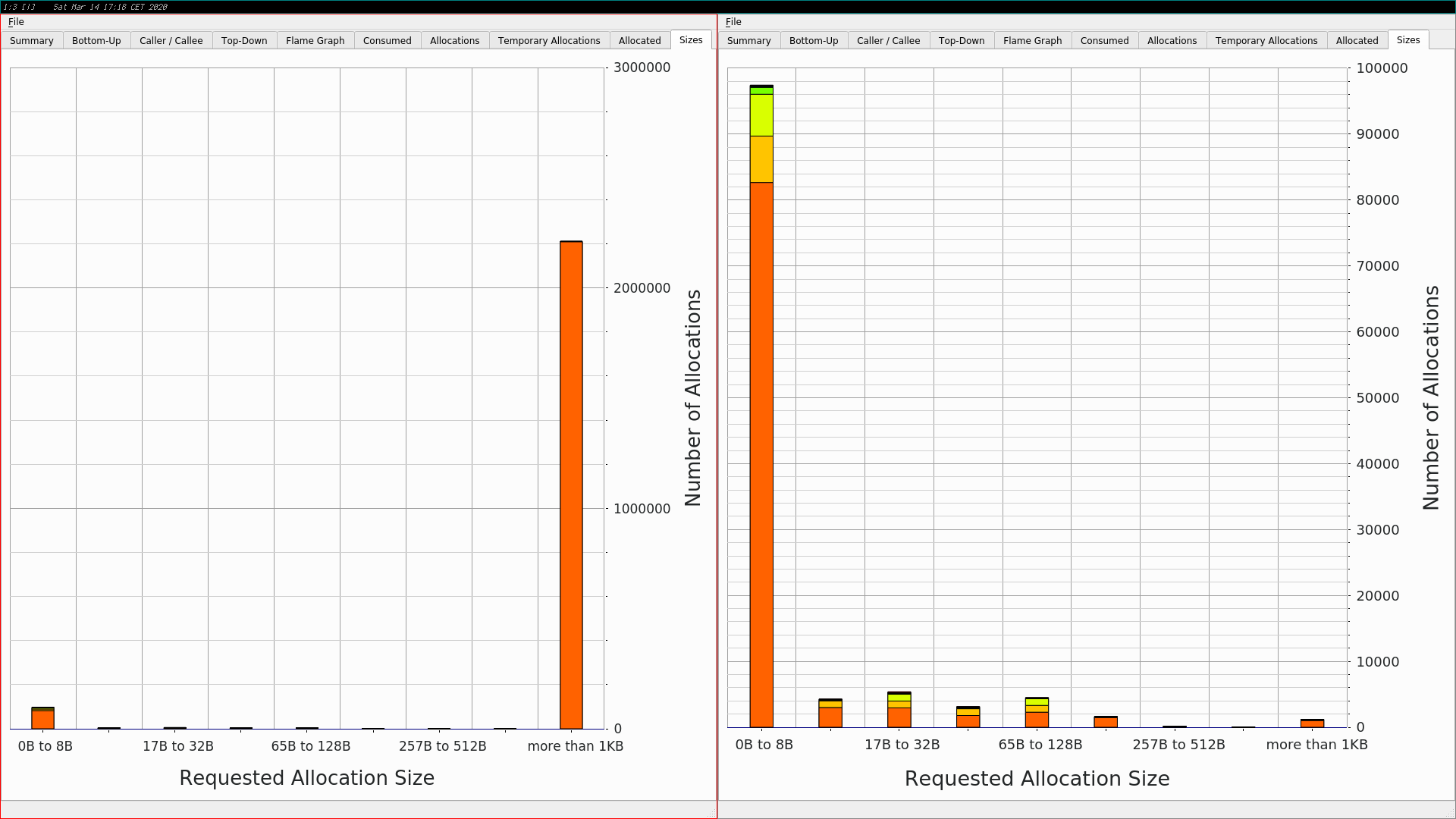For the allocated memory you can see that the lowest part (orange color) was removed on the left side, and more details of everything above it are visible on the right side.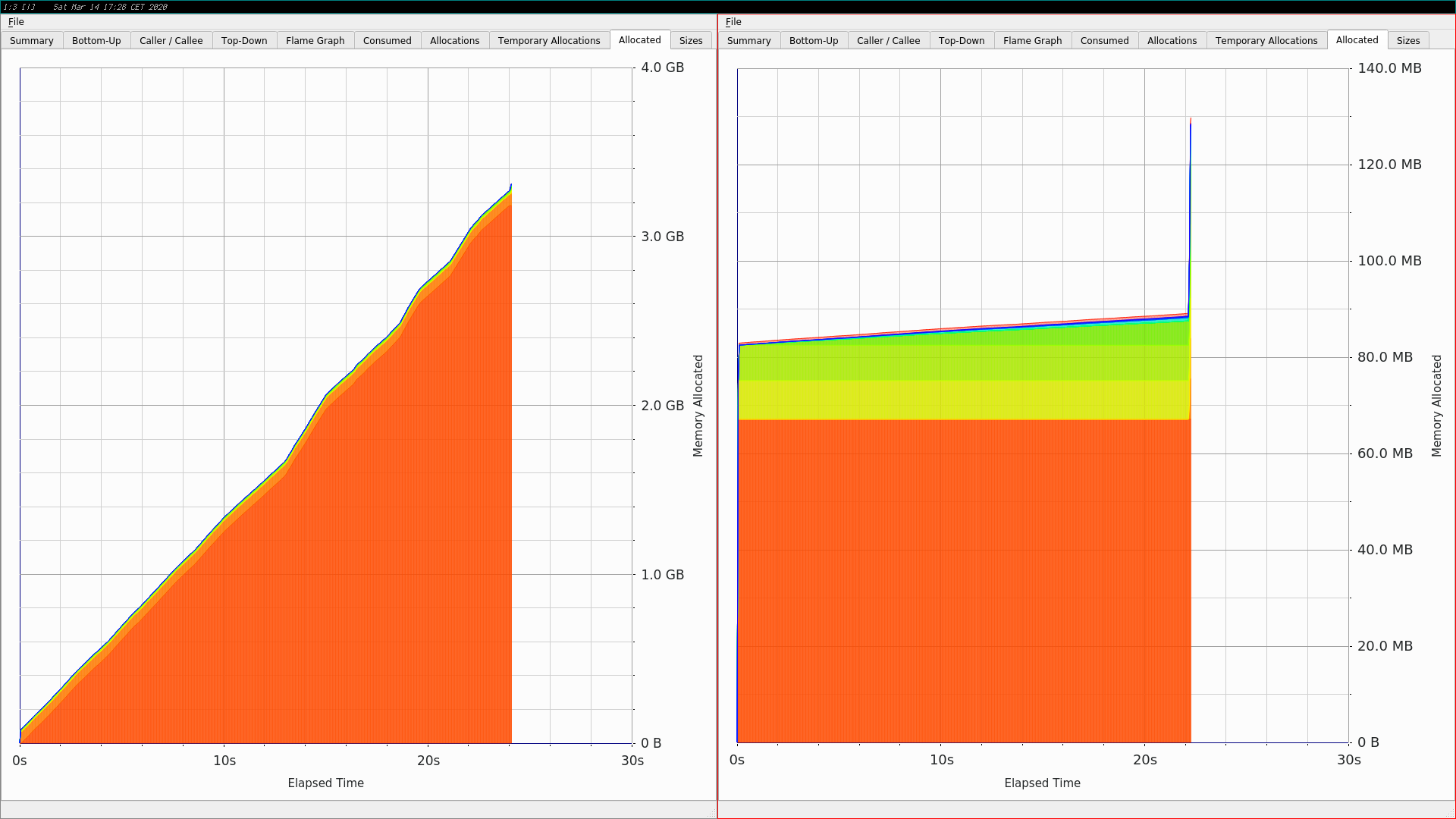## Direct Lighting

```modified   src/integrators/directlighting.rs
@@ -79,12 +78,11 @@ impl DirectLightingIntegrator {
// TODO: ProfilePhase p(Prof::SamplerIntegratorLi);
let mut l: Spectrum = Spectrum::new(0.0 as Float);
// find closest ray intersection or return background radiance
-        if let Some(mut isect) = scene.intersect(ray) {
+        let mut isect: SurfaceInteraction = SurfaceInteraction::default();
+        if scene.intersect(ray, &mut isect) {
// compute scattering functions for surface interaction
-            Rc::get_mut(&mut isect)
-                .unwrap()
-                .compute_scattering_functions(ray, false, mode);
+            isect.compute_scattering_functions(ray, false, mode);
// if (!isect.bsdf)
//     return Li(isect.SpawnRay(ray.d), scene, sampler, arena, depth);
let wo: Vector3f = isect.wo;
```

For Direct Lighting you can see as well that the new release almost entirely removes the right column.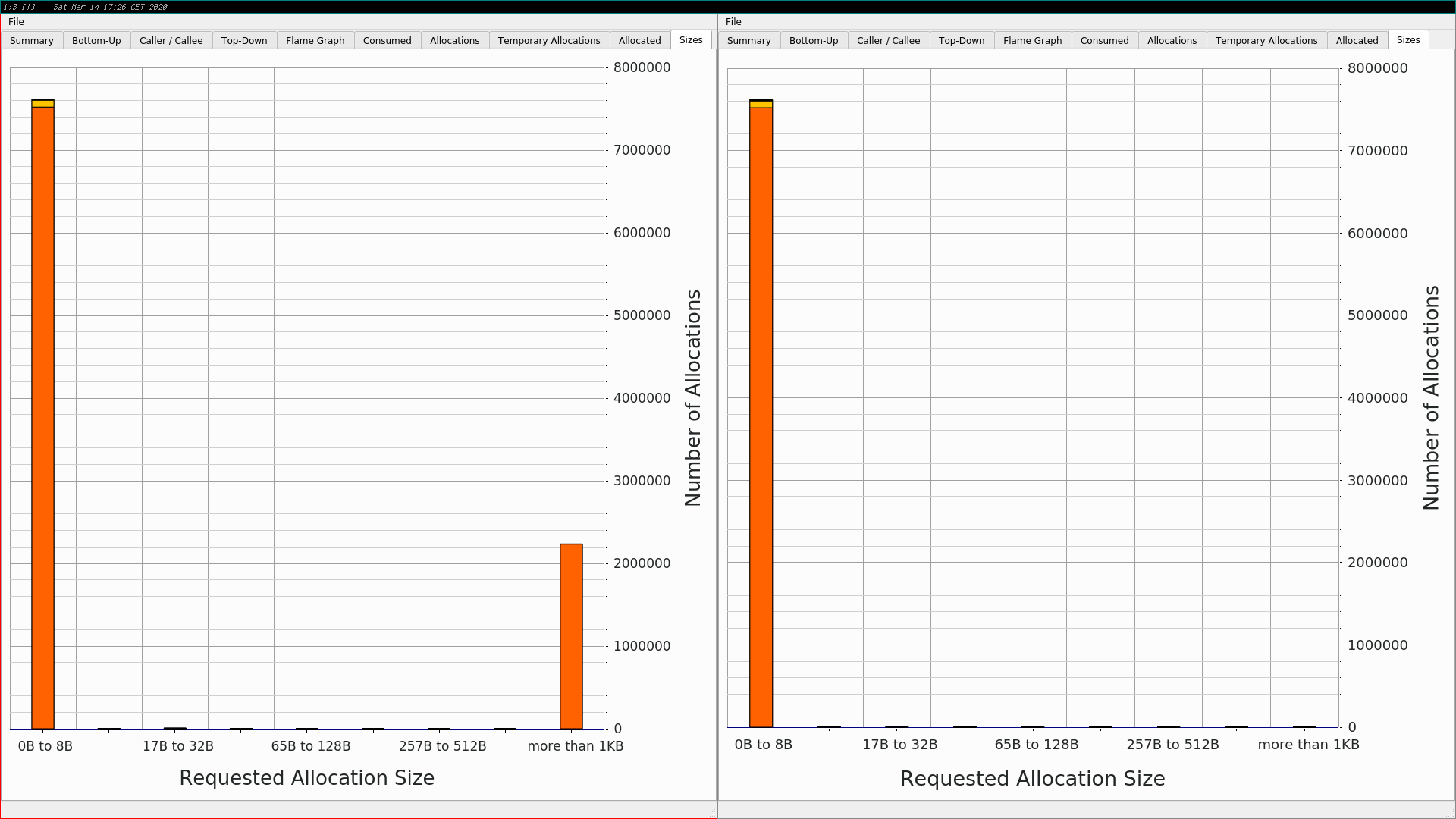The graph for the allocated memory shows as well that far less memory is allocated (because it can be re-used).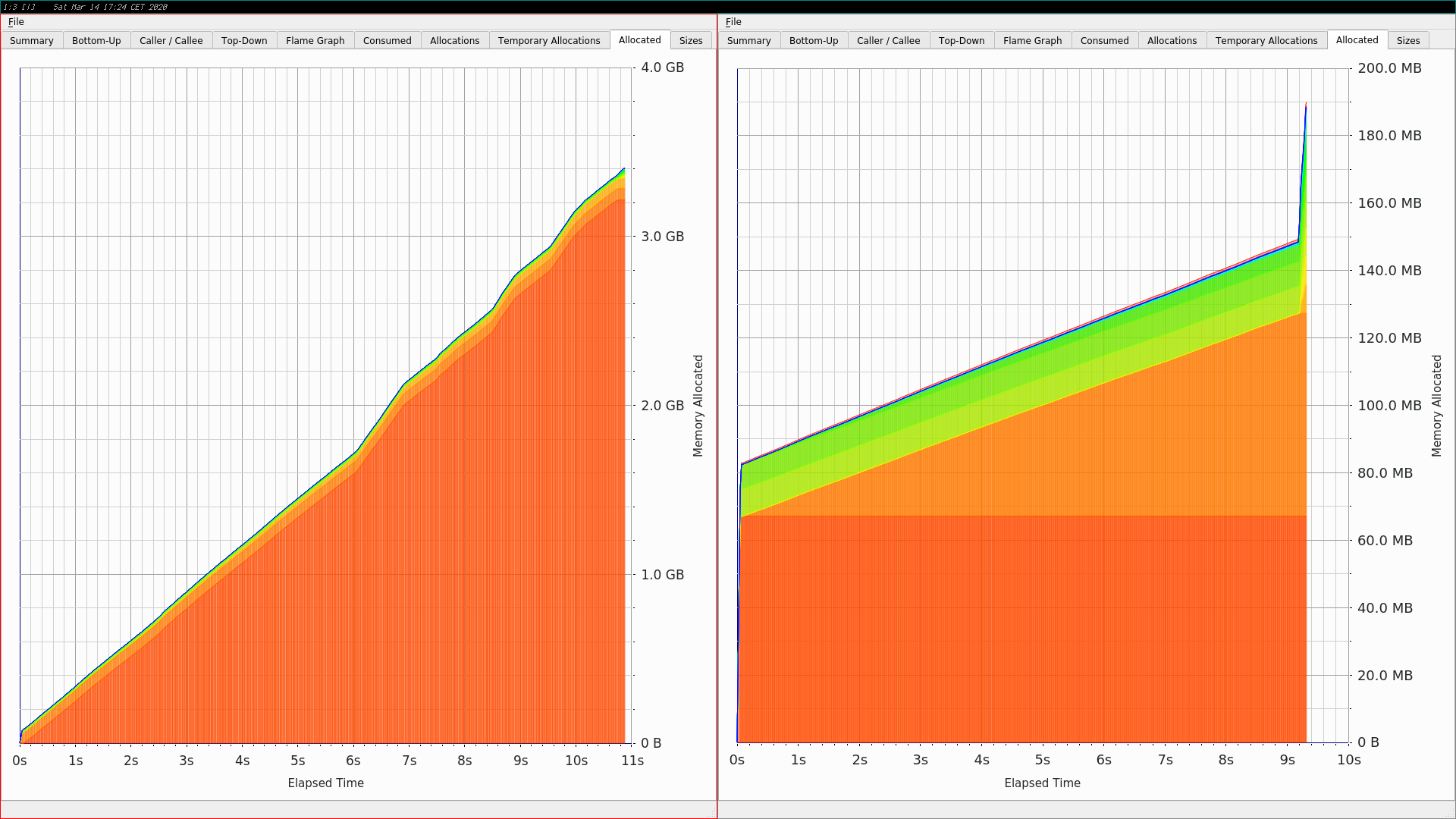## Whitted Ray Tracing

```modified   src/integrators/whitted.rs
@@ -50,7 +49,8 @@ impl WhittedIntegrator {
) -> Spectrum {
let mut l: Spectrum = Spectrum::default();
// find closest ray intersection or return background radiance
-        if let Some(mut isect) = scene.intersect(ray) {
+        let mut isect: SurfaceInteraction = SurfaceInteraction::default();
+        if scene.intersect(ray, &mut isect) {
// compute emitted and reflected light at ray intersection point

// initialize common variables for Whitted integrator
@@ -59,9 +59,7 @@ impl WhittedIntegrator {

// compute scattering functions for surface interaction
-            Rc::get_mut(&mut isect)
-                .unwrap()
-                .compute_scattering_functions(ray, false, mode);
+            isect.compute_scattering_functions(ray, false, mode);
// if (!isect.bsdf)
if let Some(ref _bsdf) = isect.bsdf {
} else {
```

For Whitted Ray Tracing the effect is similar to the one we saw for Ambient Occlusion: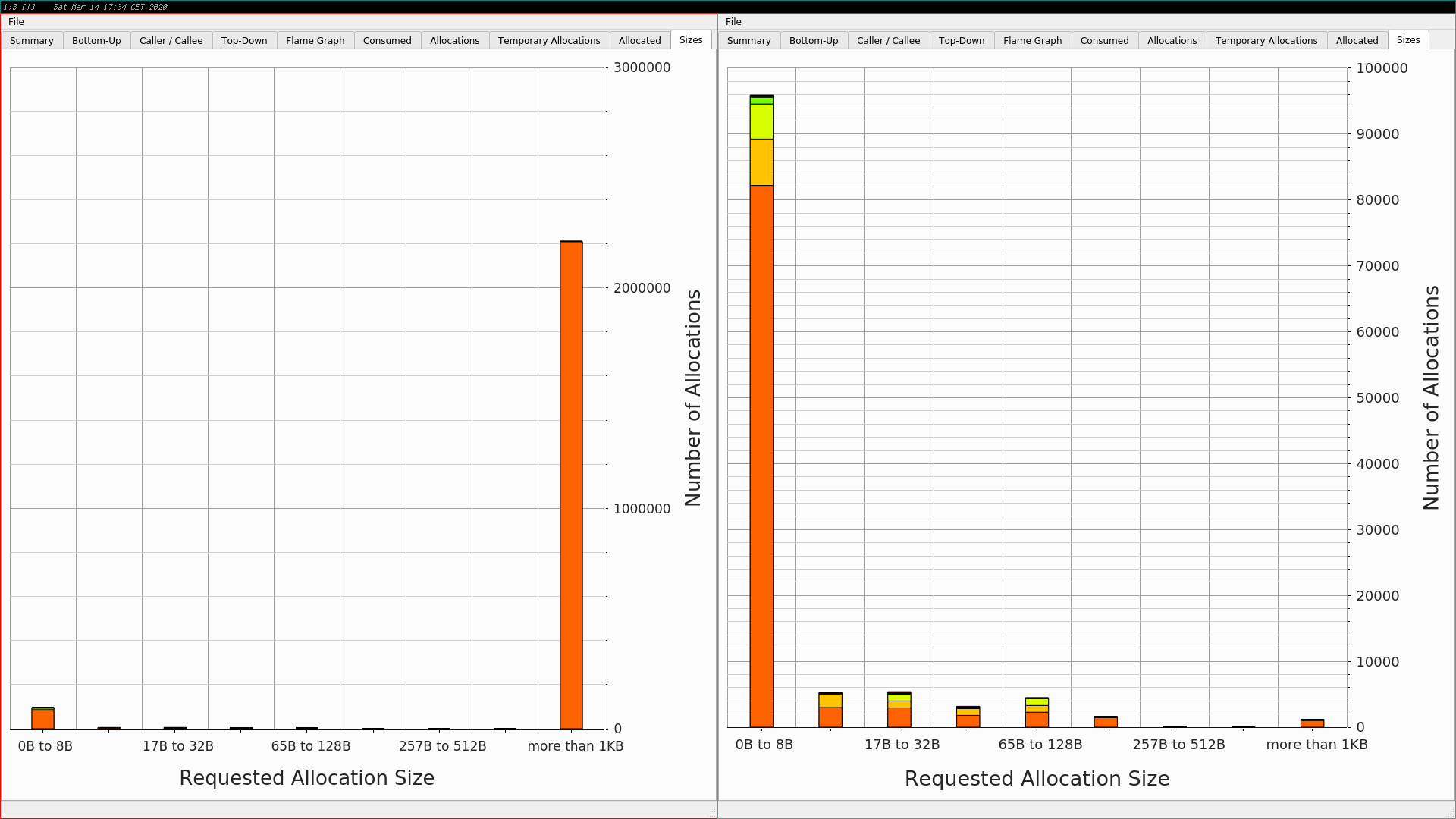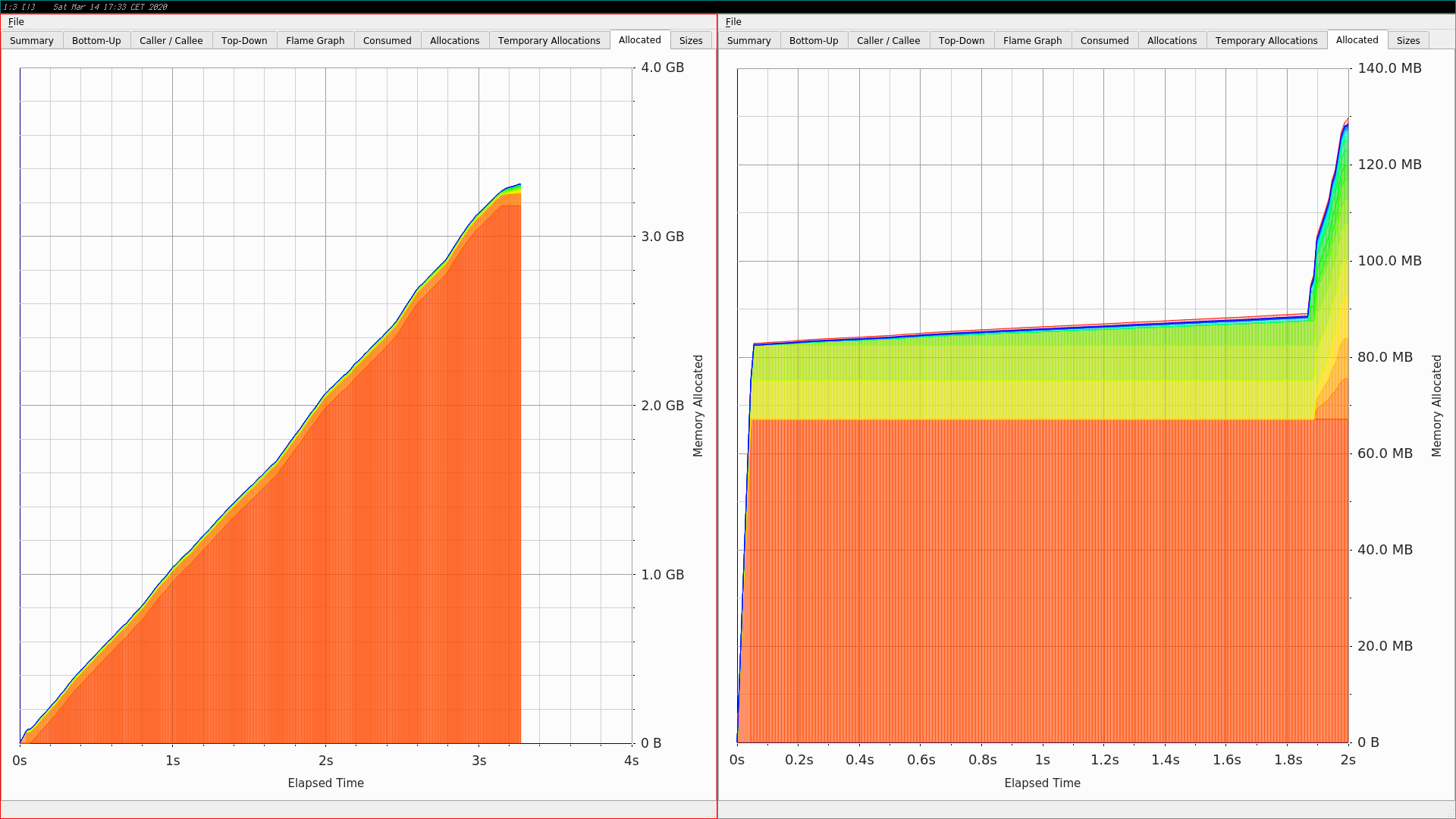## Path Tracing

```modified   src/integrators/path.rs
@@ -91,7 +90,8 @@ impl PathIntegrator {
// println!("Path tracer bounce {:?}, current L = {:?}, beta = {:?}",
//          bounces, l, beta);
// intersect _ray_ with scene and store intersection in _isect_
-            if let Some(mut isect) = scene.intersect(&mut ray) {
+            let mut isect: SurfaceInteraction = SurfaceInteraction::default();
+            if scene.intersect(&mut ray, &mut isect) {
// possibly add emitted light at intersection
if bounces == 0 || specular_bounce {
// add emitted light at path vertex
@@ -104,7 +104,7 @@ impl PathIntegrator {
}
// compute scattering functions and skip over medium boundaries
-                Rc::get_mut(&mut isect).unwrap().compute_scattering_functions(&ray, true, mode);
+                isect.compute_scattering_functions(&ray, true, mode);
if let Some(ref _bsdf) = isect.bsdf {
// we are fine (for below)
} else {
```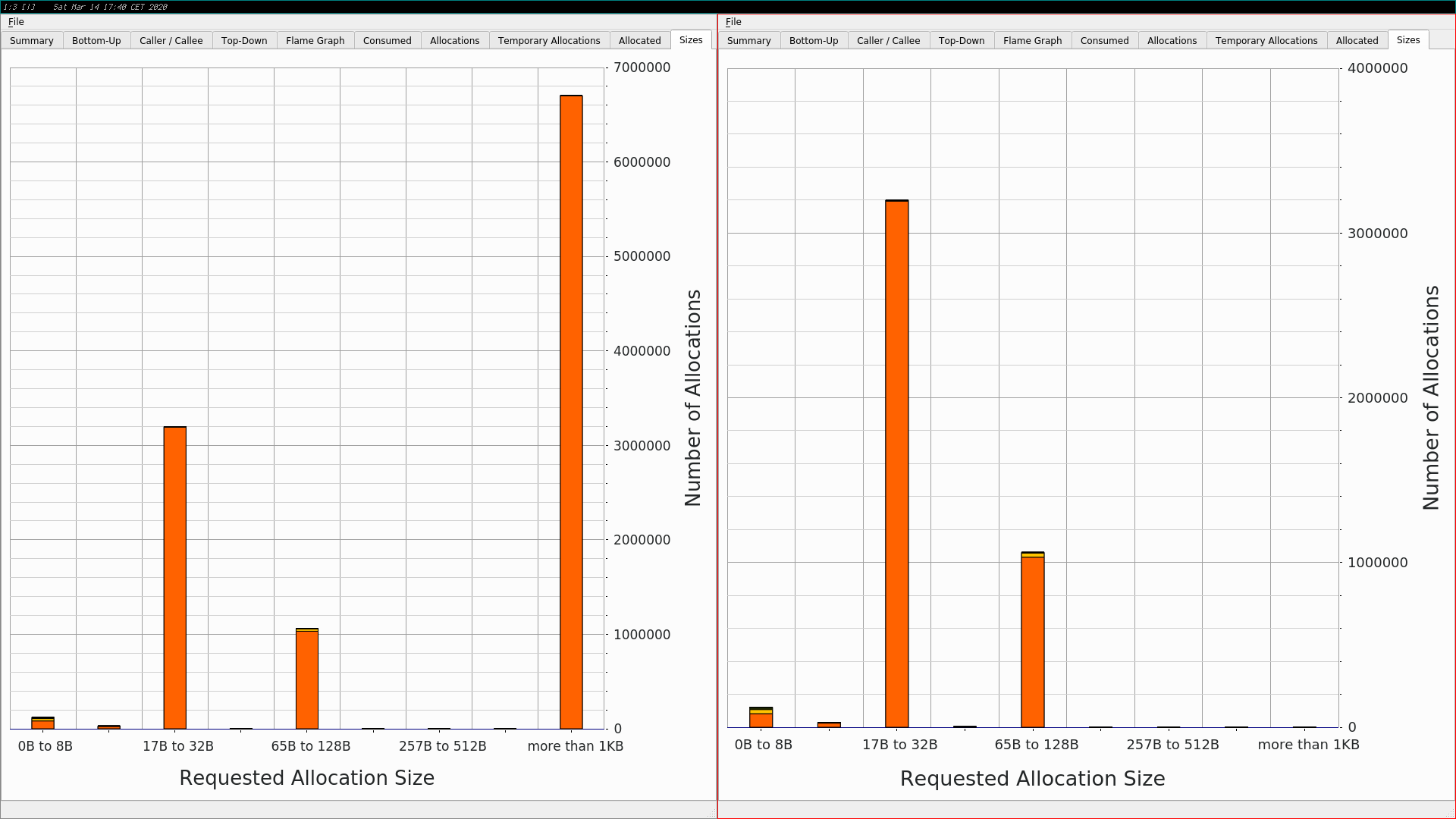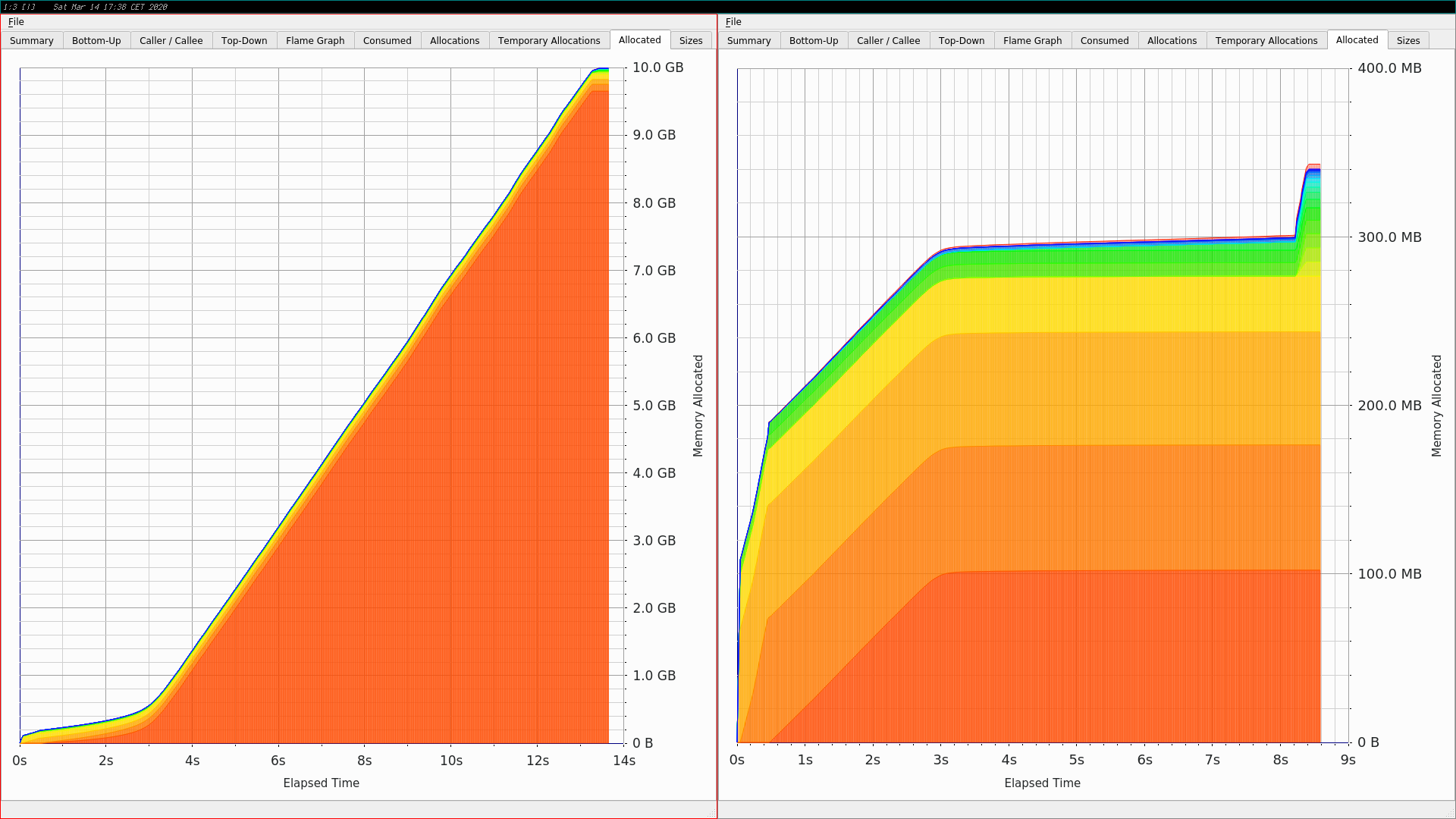## Bi-Directional Path Tracing

```modified   src/integrators/bdpt.rs
@@ -1218,11 +1221,10 @@ pub fn random_walk<'a>(
//     bounces, beta, pdf_fwd, pdf_rev
// );
let mut mi_opt: Option<MediumInteraction> = None;
-        let mut si_opt: Option<Rc<SurfaceInteraction>> = None;
// trace a ray and sample the medium, if any
let found_intersection: bool;
-        if let Some(isect) = scene.intersect(&mut ray) {
-            si_opt = Some(isect);
+        let mut isect: SurfaceInteraction = SurfaceInteraction::default();
+        if scene.intersect(&mut ray, &mut isect) {
found_intersection = true;
} else {
found_intersection = false;
@@ -1286,13 +1288,10 @@ pub fn random_walk<'a>(
bounces += 1;
}
break;
-            }
-            if let Some(mut isect) = si_opt {
+            } else {
// compute scattering functions for _mode_ and skip over medium
// boundaries
-                Rc::get_mut(&mut isect)
-                    .unwrap()
-                    .compute_scattering_functions(&ray, true, mode);
+                isect.compute_scattering_functions(&ray, true, mode);
let isect_wo: Vector3f = isect.wo;
if isect.bsdf.is_none() {
```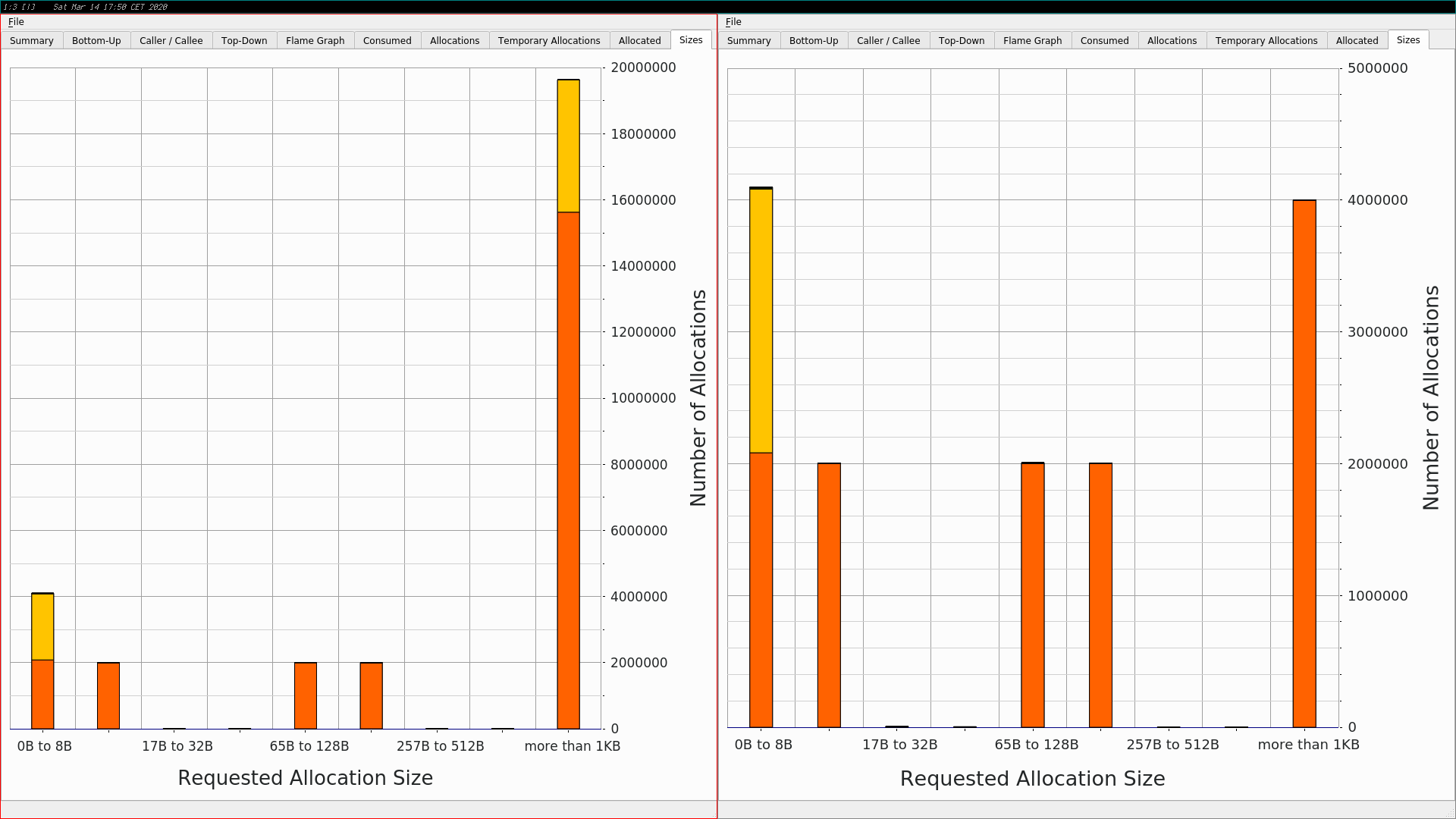## Metropolis Light Transport

```modified   src/integrators/mlt.rs
@@ -437,7 +437,9 @@ impl MLTIntegrator {
) * (n_strategies as Float)
}
pub fn render(&self, scene: &Scene, num_threads: u8) {
-        let num_cores = if num_threads == 0_u8 {
+        let mut num_cores: usize; // TMP
+        // let num_cores = if num_threads == 0_u8 {
+        let num_cores_init = if num_threads == 0_u8 { // TMP
num_cpus::get()
} else {
@@ -445,6 +447,7 @@ impl MLTIntegrator {
if let Some(light_distr) = compute_light_power_distribution(scene) {
println!("Generating bootstrap paths ...");
// generate bootstrap samples and compute normalization constant \$b\$
+            num_cores = 1; // TMP: disable multi-threading
let n_bootstrap_samples: u32 = self.n_bootstrap * (self.max_depth + 1);
let mut bootstrap_weights: Vec<Float> =
vec![0.0 as Float; n_bootstrap_samples as usize];
@@ -477,7 +480,13 @@ impl MLTIntegrator {
)));
let mut p_raster: Point2f = Point2f::default();
*weight = integrator
-                                        .l(scene, &light_distr, &mut sampler, depth, &mut p_raster)
+                                        .l(
+                                            scene,
+                                            light_distr.clone(),
+                                            &mut sampler,
+                                            depth,
+                                            &mut p_raster,
+                                        )
.y();
}
});
@@ -499,6 +508,7 @@ impl MLTIntegrator {
let bootstrap: Distribution1D = Distribution1D::new(bootstrap_weights);
let b: Float = bootstrap.func_int * (self.max_depth + 1) as Float;
// run _n_chains_ Markov chains in parallel
+            num_cores = num_cores_init; // TMP: re-enable multi-threading
let film: Arc<Film> = self.get_camera().get_film();
let n_total_mutations: u64 =
self.mutations_per_pixel as u64 * film.get_sample_bounds().area() as u64;
```

For the Metropolis Light Transport algorithm most of the changes were done in the file responsible for Bi-Directional Path Tracing because they both share some functionality. But what's important is that I had to (temporarily) disable multi-threading for a phase where bootstrap samples are generated and a normalization constant is computed. I might be able to activate multi-threading (for this particular phase) again in later releases, but for now it was more important to save some time for almost all algorithms by re-using the struct `SurfaceInteraction` where possible instead of allocating and dropping the memory all the time.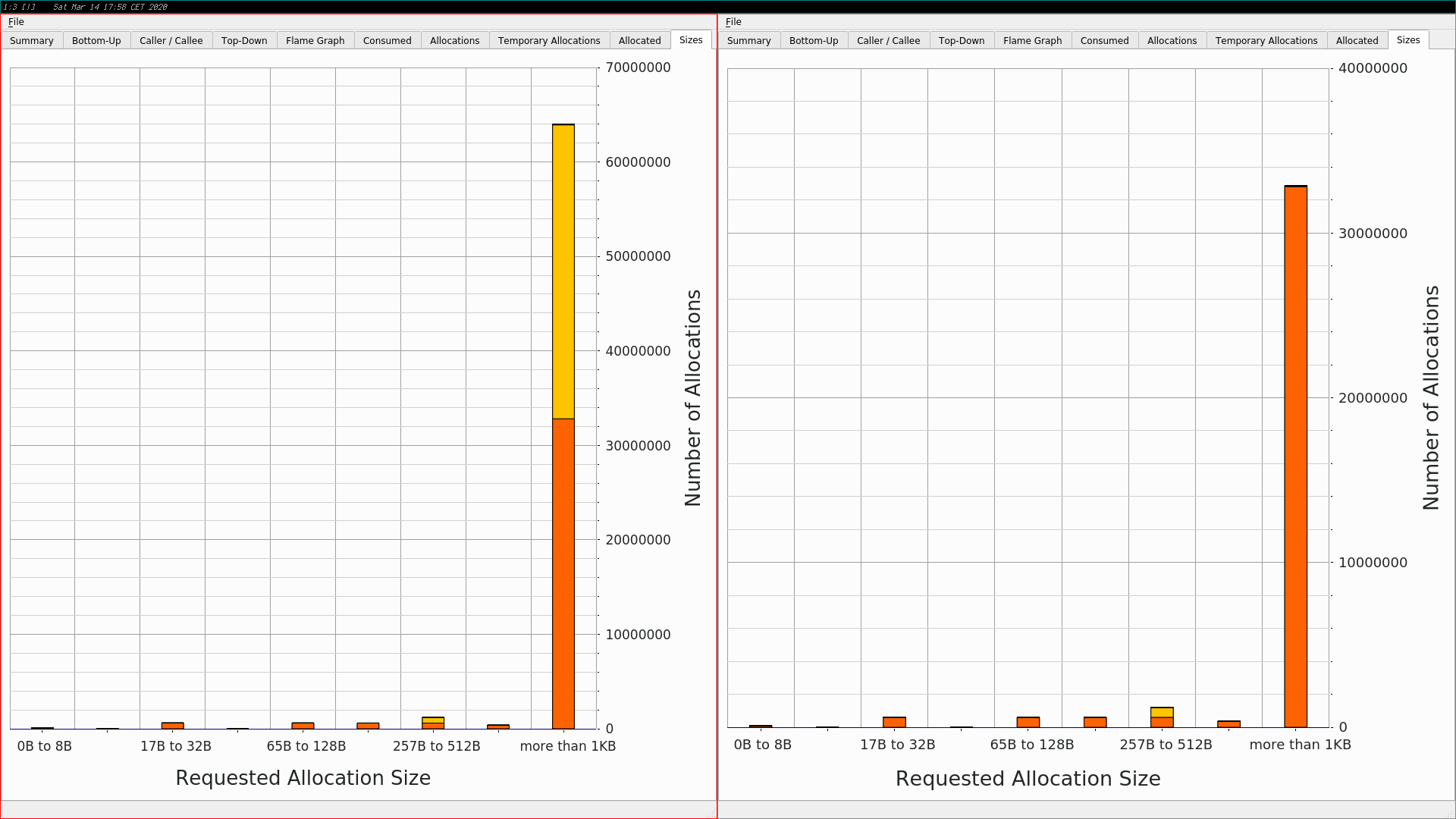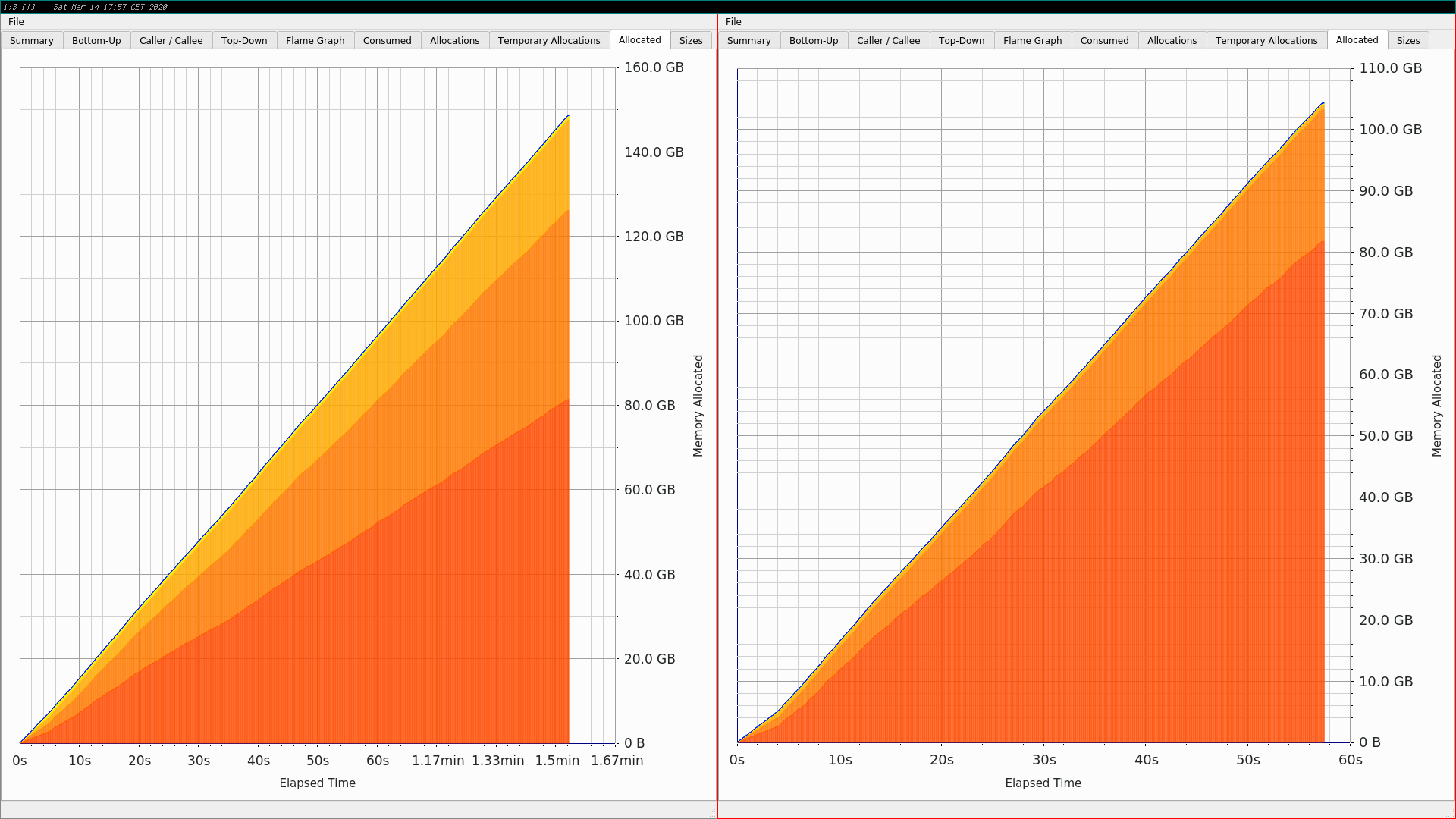## Unsafe Code

If you look at the change to the struct `SurfaceInteraction` again:

```modified   src/core/interaction.rs
@@ -274,7 +274,7 @@ pub struct SurfaceInteraction<'a> {
pub dvdx: Cell<Float>,
pub dudy: Cell<Float>,
pub dvdy: Cell<Float>,
-    pub primitive: Option<&'a Primitive>,
+    pub primitive: Option<*const Primitive>,
pub bsdf: Option<Bsdf>,
pub bssrdf: Option<TabulatedBssrdf>,
```

The new release allocates the memory for the struct `SurfaceInteraction` when a ray hits a shape, but the pointer to a `Primitive` will be set later (or better: somewhere else). Therefore we have to use an `Option` because we can't use a `NULL` pointer (like in C++).

```\$ rg -trust "\.primitive = None"
src/shapes/triangle.rs
453:        isect.primitive = None;
...
```

So here are some places where that missing information is filled in:

```\$ rg -trust "\.primitive = Some"
...
src/core/primitive.rs
43:                    isect.primitive = Some(self);
...
src/integrators/bdpt.rs
1326:                    si_eval.primitive = Some(primitive);
```

```Recall that we can create raw pointers in safe code, but we can’t
dereference raw pointers and read the data being pointed to.
```

Therefore we need unsafe code to access the `Primitive` pointed to:

```\$ rg -trust "primitive = unsafe"
src/core/scene.rs
99:		let primitive = unsafe { &*primitive_raw };

src/core/interaction.rs
426:	    let primitive = unsafe { &*primitive_raw };
516:	    let primitive = unsafe { &*primitive_raw };

src/core/integrator.rs
543:                        let primitive = unsafe { &*primitive_raw };
556:                    let primitive = unsafe { &*primitive_raw };

src/core/light.rs
214:		    let primitive = unsafe { &*primitive_raw };

src/integrators/bdpt.rs
472:                    let primitive = unsafe { &*primitive_raw };
538:                let primitive = unsafe { &*primitive_raw };
657:                    let primitive = unsafe { &*primitive_raw };
746:                        let primitive = unsafe { &*primitive_raw };
```

Here is a piece of Rust code how to get access to the `Primitive` from the `SurfaceInteraction` and call a member function:

```if hit_surface {
found_surface_interaction = true;
if let Some(primitive_raw) = light_isect.primitive {
let primitive = unsafe { &*primitive_raw };
if let Some(area_light) = primitive.get_area_light() {
...
}
}
}
```

## The End

I hope I didn't forget anything important. Have fun and enjoy the v0.8.1 release.